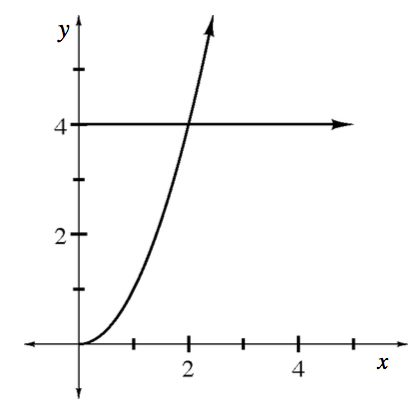### Home > APCALC > Chapter 8 > Lesson 8.2.3 > Problem8-96

8-96.

Multiple Choice: The function $f (x) = x^2$ is bounded by the $y$-axis and the line $y = 4$. The volume of the solid generated by revolving the region about the $y$-axis can be computed by which of the following integrals?

1. $2 \pi \int _ { 0 } ^ { 2 } x ( 4 - x ^ { 2 } ) d x$

1. $\pi \int _ { 0 } ^ { 4 } y d y$

1. $\pi \int _ { 0 } ^ { 4 } \sqrt { y } d y$

 A. I only B. II only C. III only D. I and II E. I and IIIIf you want to use disks/washers, then rotate horizontal rectangles.
This means that both the bounds and the integrand must be written in terms of $y$.

$\text{Disks: }\pi \int_{y=a}^{y=b}(f(y))^{2}dy$

$\text{Washers: }\pi \int_{y=a}^{y=b}(f(y))^{2}-(g(y))^{2}dy$

If you want to use shells, then use $x$-values.

$\text{Shells: }2\pi \int_{x=a}^{x=b}(x)f(x)dx$